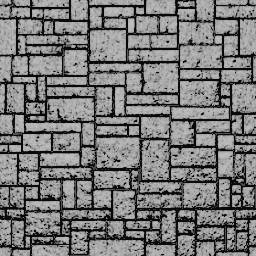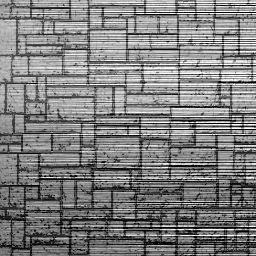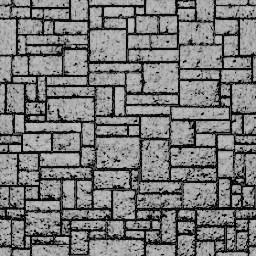# Reconstructing a height map from a normal map

At times it happens that we have the normal map but not the height/displacement map that was used to create it. For some rendering techniques, like parallax mapping, it is the height map which is needed. In addition, having the height map is beneficial for editing the normal map.A height map and its normal map, used in the samples below.

Recovering the height map boils down to integrating the normal map. This is what is suggested in multiple places around the web (e.g. here, here and here), but it is not explained how to do this in practice.

The problem is that the normal map at hand is typically encoded with low precision, 8-bits per channel usually, and might be compressed with a lossy algorithm afterwards. In the end, the line integrals along closed curves aren’t zero, and so the end result significantly depends on the curves along which we integrate. In fact, no matter how we integrate, we end up with significant noise in the output.Different integration paths give different results. None are good.

## Through deconvolution

Denote by f(x, y) the height map and n(x, y) = (nx, ny, 1) the normal map that was calculated by some finite difference scheme, that is by convolving the original height map with the kernels dx and dy. For the sake of boundary condition, we assume that both f and n are periodic of periods w and h. We seek a function f which minimizes:

E = 2−1 ( ǁdx ∗ f − nxǁ2 + ǁdy ∗ f − nyǁ2 )

Taking the Fourier transform F, Dx, Dy, Nx, Ny of f, dx, dy, nx, ny respectively, and applying Parseval's theorem:

E = 2−1 ( ǁDx F − Nxǁ2 + ǁDy F − Nyǁ2 )

The last expression can be minimized by each F(x,y) independently. The minimum is achieved by:

F = (|Dx|2 + |Dy|2)−1 (conj(Dx) Nx + conj(Dy) Ny)

For when |Dx|2 + |Dy|2 = 0, E is independent of F(x,y), and so we can choose any value of the later. In such cases we seek the minimum norm solution, which is achieved by setting F = 0 at these points.

Applying the inverse Fourier transform to F leads to the minimum error, minimum norm solution f.The reconstructed height map. Running time: < 1 ms

## Implementation

A command line utility implementing the above is available at debump.c.